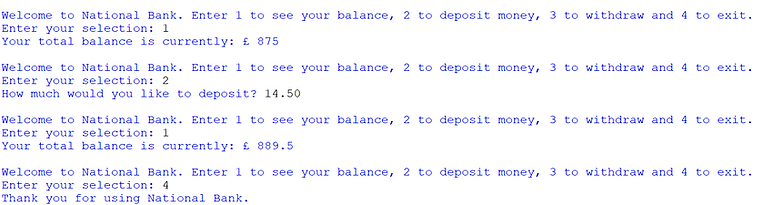top of page
top

## Python 7b - Functions## What is a Function?

A function is a subroutine that takes one or more values from the main program and returns a value back.

For example, transferring over a sphere’s radius from the main program for the function to calculate a surface area and then return that value to the main program.

The two key differences between procedures and functions are:

1. A function uses parameters to transfer data from the main program into the function.

2. A function returns a value to the main program.

## Writing Functions

A function is written the same way as a procedure but it uses parameters.

In the example below the parameters are num1 and num2 which are sent from the main program to be used in the function.

The return command is used to send a value back to the main program.Below is another example of a function that takes the radius of a sphere and works out the area in a separate function.

The area is returned to the main program and printed.Subroutines can be reused and called with different parameters.

The program below repeatedly takes an integer input and adds it to a total in a function that is then returned and printed.Create a program similar to the sphere example above, this time to work out the volume of a cylinder.

In the main program ask the user to enter the cylinder's radius and then its height.

The actual calculation should be done in a function and returned to the main program.

The calculation for a cylinder's volume is:

Extension: Use the round command from section 9b to round the number to 2 decimal places.

Example solution:## Using Subroutines as a Menu

Subroutines are often used to split programs up and give users a selection of options.

Subroutines are used for this purpose because they are separate, making it easier to code and manage a program.

The example below for a simplified online banking system uses separate subroutines accessible within a while true loop.

Depending on the option chosen by the user, the appropriate subroutine will be called.Instead of a further practice task here, Task 4 of the Section 7 Practice tasks page challenges you to make a similar program using multiple subroutines.

bottom of page